The Theory \ Details \ parameters estimation \ sample estimates

# Model Parameters Estimation

## Maximum likelihood estimates for means and covariances

Consider segmentwith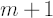observations of the discounted prices: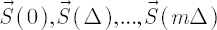, where. Proceeding from practical reasons, we shall assume that the above prices don’t take into account the dividend yields and the risk-free rate. Based on the prices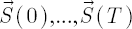one forms the range of logarithmic returns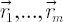of length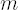by means of the formula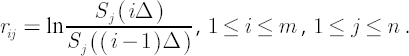### Sample Expected Growth Rate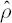The MLE for expected growth rate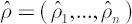is the annualized average of.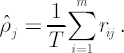Sample estimate for expected excess growth rate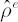iswhere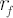denotes risk-free rate;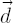denotes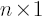vector of dividend yields;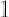stands forvector of ones.

### Sample Covariance matrix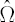The Covariance matrix estimate, calculated in SmartFolio, is an annualized sample covariance matrixof the range.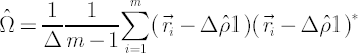### Sample Mu vectorTo estimateone should resort to the following expression: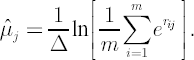Note. If price process satisfies the analytical model assumptions, then sample Mu vectorcan be calculated in alternative way from a sample expected growth rateand a sample covariance matrix: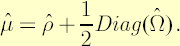Corresponding sample estimatefor excess Mu has the following form: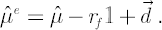Copyright © 2006-2019 Modern Investment Technologies